Function to generate a color legend, the legend may be added to an existing plot or drawn in a separate plotting window.

colorlegend(color = NULL, ncol = NULL, x = NULL, breaks = NULL,
pos = "center", shift = 0.02, side.legend = 1L, side.ticks = 1L,
range = NULL, lrange = NULL, width = 0.25, height = 0.05,
scale = TRUE, xlim = NULL, ylim = NULL, plot = NULL, full = FALSE,
add = FALSE, col.border = "black", lty.border = 1L, lwd.border = 1L,
ticks = TRUE, at = NULL, col.ticks = "black", lwd.ticks = 1L,
lty.ticks = 1L, length.ticks = 0.3, labels = NULL,
distance.labels = 0, col.labels = "black", cex.labels = 1L,
digits = 2L, swap = FALSE, symmetric = TRUE, xpd = NULL,
title = NULL, side.title = 2, shift.title = c(0, 0),
cex.title = 1, ...)

## Arguments

color Character, integer. The colors for the legend, may also be a function, e.g. colors = heat.colors. Integer, the number of different colors that should be generated if color is a function. Numeric, values for which the color legend should be drawn. Numeric, a set of breakpoints for the colors: must give one more breakpoint than ncol. Character, numeric. The position of the legend. Either a numeric vector, e.g. pos = c(0.1, 0.2) will add the legend at the 10$$\%$$ point in the x-direction and at the 20$$\%$$ point in the y-direction of the plotting window, may also be negative, or one of the following: "bottomleft", "topleft", "topright", "bottomright", "left", "right", "top", "bottom" and "center". Numeric, if argument pos is a character, shift determines the distance of the legend from the plotting box. Integer, if set to 2 the legend will be flipped by 90 degrees. Integer, if set to 2, the ticks and labels will be on the opposite site of the legend. Numeric, specifies a range for x values for which the legend should be drawn. Numeric, specifies the range of legend. Numeric, the width of the legend, if scale = TRUE the width is proportional to the x-limits of the plotting window. Numeric, the height of the legend, if scale = TRUE the height is proportional to the y-limits of the plotting window. Logical, if set to TRUE, the width and height of the legend will be calculated proportional to the x- and y-limits of the plotting window. Numeric, the x-limits of the plotting window the legend should be added for, numeric vector, e.g., returned from function range. Numeric, the y-limits of the plotting window the legend should be added for, numeric vector, e.g., returned from function range. Logical, if set to TRUE, the legend will be drawn in a separate plotting window. Logical, if set to TRUE, the legend will be drawn using the full window range. Logical, if set to TRUE, the legend will be added to an existing plot. The color of the surrounding border line of the legend. The line type of the surrounding border line of the legend. The line width of the surrounding border line of the legend. Logical, if set to TRUE, ticks will be added to the legend. Numeric, specifies at which locations ticks and labels should be added. The colors of the ticks. The line width of the ticks. The line type of the ticks. Numeric, the length of the ticks as percentage of the height or width of the colorlegend. Character, specifies labels that should be added to the ticks. Numeric, the distance of the labels to the ticks, proportional to the length of the ticks. The colors of the labels. Text size of the labels. Integer, the decimal places if labels are numerical. Logical, if set to TRUE colors will be represented in reverse order. Logical, if set to TRUE, a symmetric legend will be drawn corresponding to the +- max(abs(x)) value. Sets the xpd parameter in function par. Character, a title for the legend. Integer, 1 or 2. Specifies where the legend is placed, either on top if side.title = 1 or at the bottom if side.title = 2. Numeric vector of length 2. Specifies a possible shift of the title in either x- or y-direction. Text size for the title. Other graphical parameters to be passed to function text.

## Value

A named list with the colors generated, the breaks and the function map, which may be used for mapping of x values to the colors specified in argument colors, please see the examples below.

## Examples

## Play with colorlegend.
colorlegend()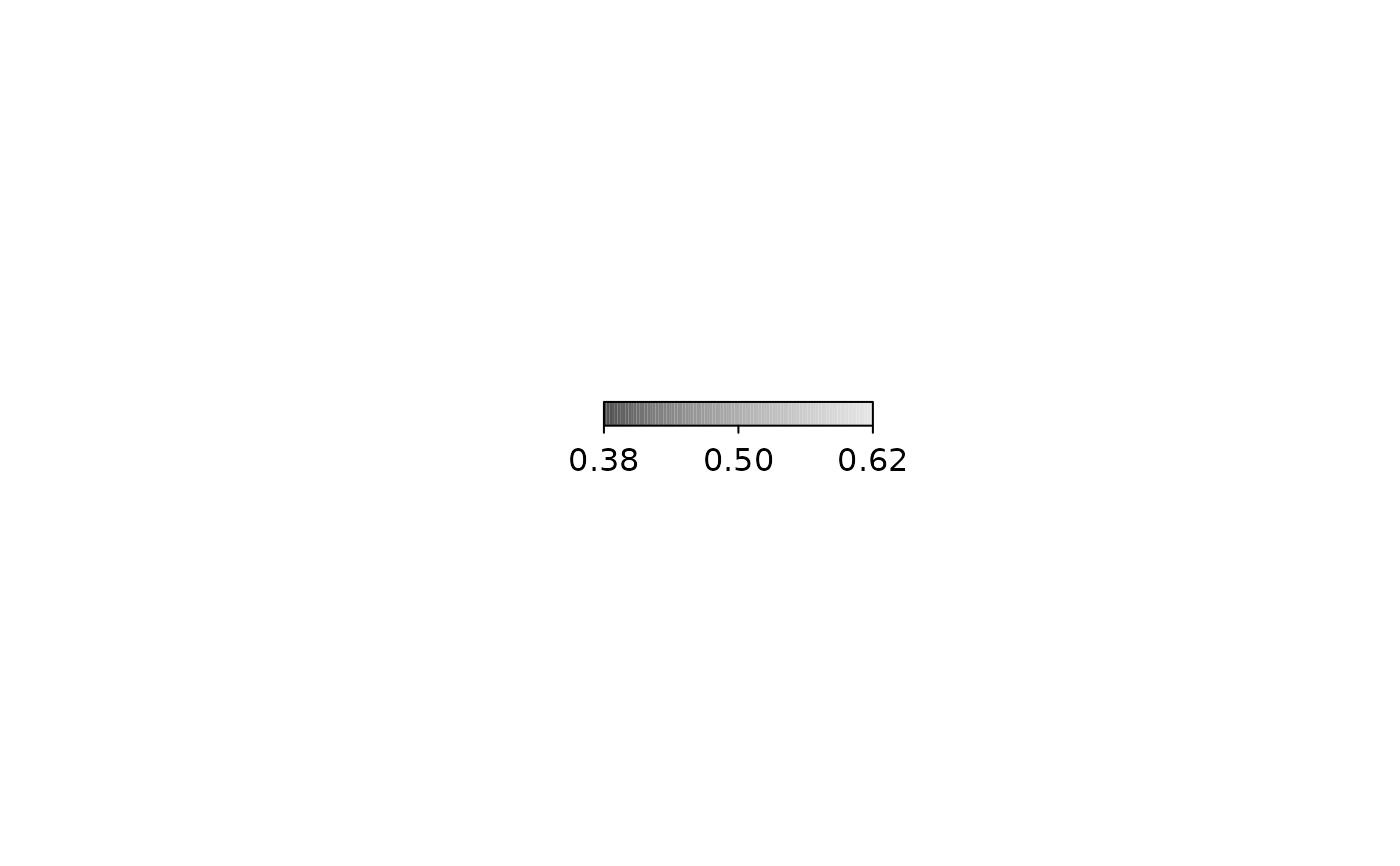colorlegend(side.legend = 2)colorlegend(side.legend = 2, side.ticks = 2)colorlegend(height = 2)colorlegend(width = 1, height = 0.8, scale = FALSE,
pos = c(0, 0.2), length.ticks = 0.5)colorlegend(color = heat.colors, ncol = 9)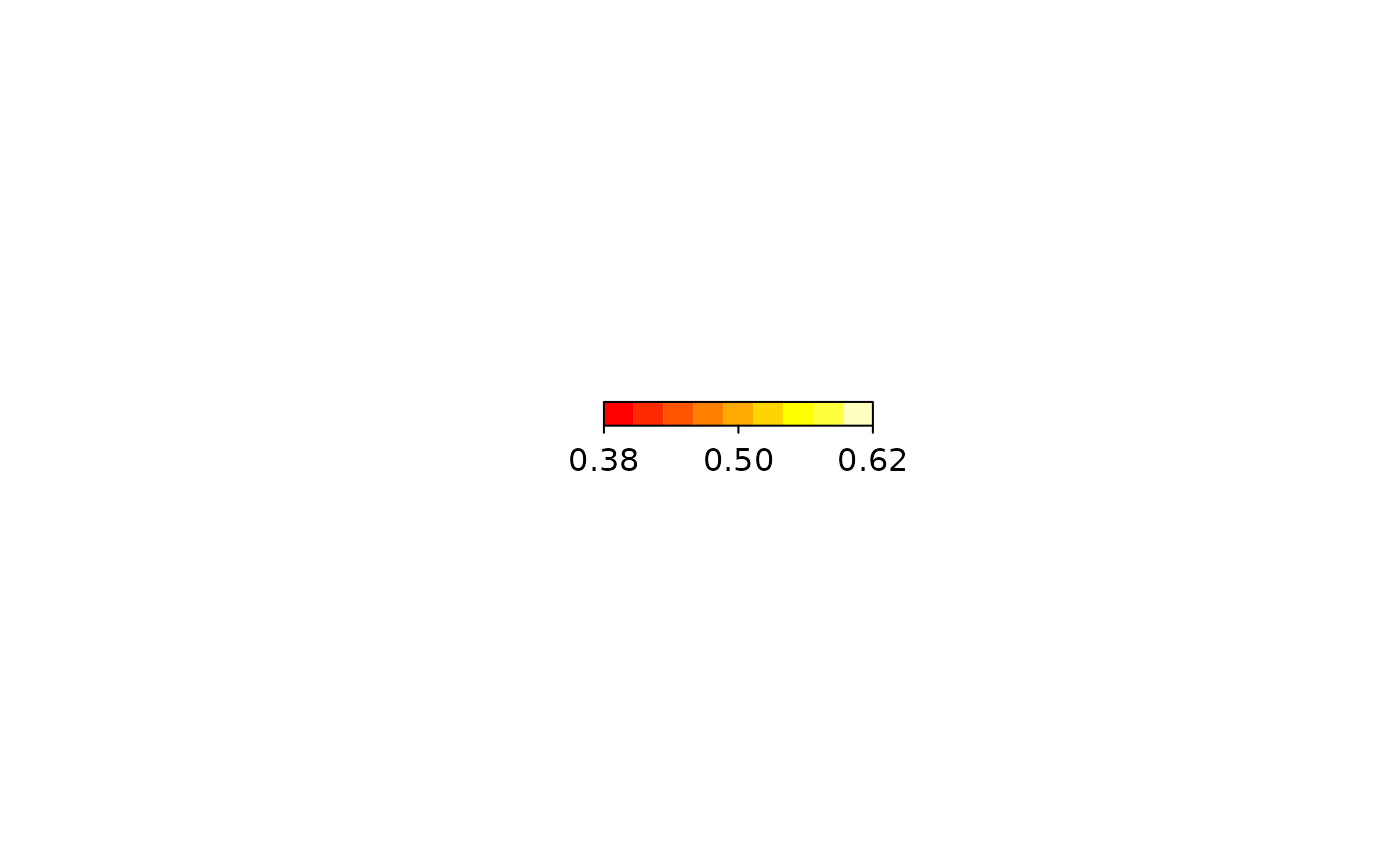colorlegend(color = heat.colors, ncol = 9, swap = TRUE)colorlegend(pos = "bottomleft")colorlegend(pos = "topleft")colorlegend(pos = "topright")colorlegend(pos = "bottomright")## Take x values for the color legend.
x <- runif(100, -2, 2)
colorlegend(color = diverge_hcl, x = x)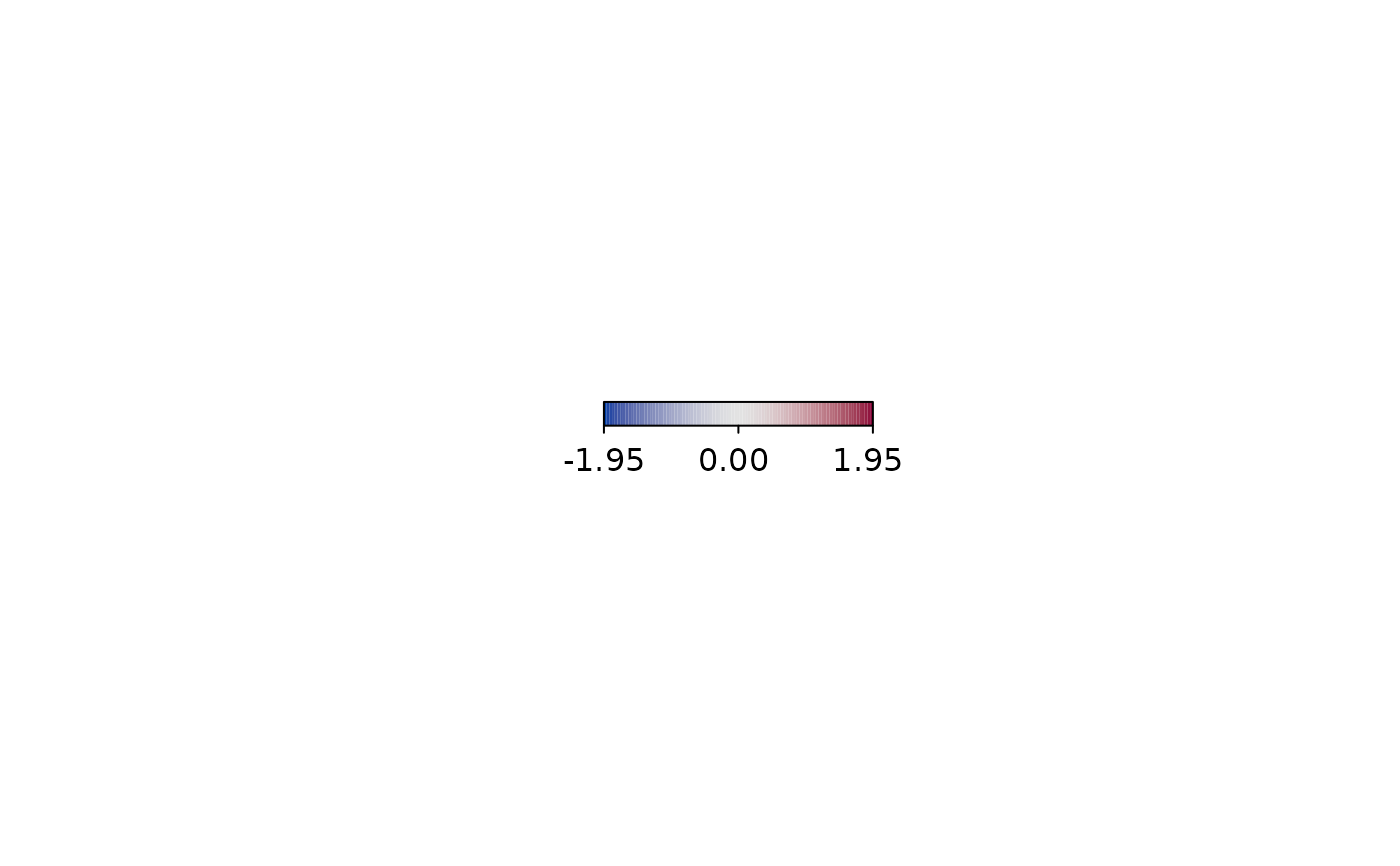colorlegend(color = diverge_hcl, x = x, at = c(-1.5, 0, 1.5))colorlegend(color = diverge_hcl, x = x, at = c(-1.5, 0, 1.5),
labels = c("low", "middle", "high"))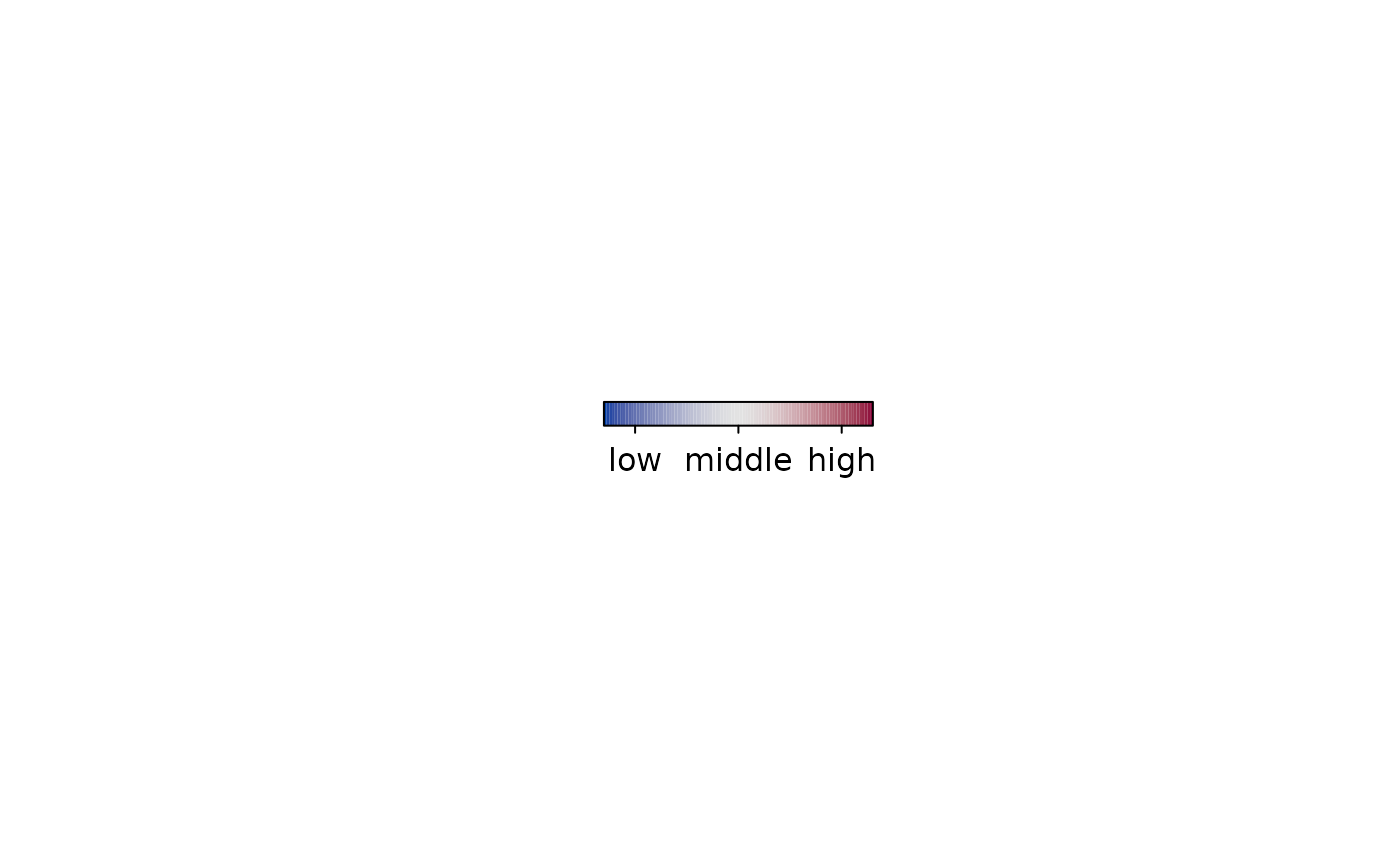colorlegend(color = rainbow_hcl, x = x, at = c(-1.5, 0, 1.5),
labels = c("low", "middle", "high"), length.ticks = 1.5)colorlegend(color = heat_hcl, x = x, at = c(-1.5, 0, 1.5),
labels = c("low", "middle", "high"), length.ticks = 1.5,
lwd.border = 2, lwd.ticks = 2, cex.labels = 1.5, font = 2)colorlegend(color = topo.colors, x = x, at = c(-1.5, 0, 1.5),
labels = c("low", "middle", "high"), length.ticks = 1.5,
lwd.border = 2, lwd.ticks = 2, cex.labels = 1.5, font = 2,
col.border = "green3", col.ticks = c(2, 5, 2),
col.labels = c(6, 4, 3))colorlegend(color = diverge_hsv, x = x, at = c(-1.5, 0, 1.5),
labels = c("low", "middle", "high"), length.ticks = 1.5,
lwd.border = 2, lwd.ticks = 2, cex.labels = 1.5, font = 2,
col.border = "green3", col.ticks = c(2, 5, 2),
col.labels = c(6, 4, 3), lty.border = 2, lty.ticks = c(2, 3, 2))colorlegend(color = diverge_hsv, x = x, at = c(-1.5, 0, 1.5),
labels = c("low", "middle", "high"), length.ticks = 1.5,
lwd.border = 2, lwd.ticks = 2, cex.labels = 1.5, font = 2,
col.border = "green3", col.ticks = c(2, 5, 2),
col.labels = c(6, 4, 3), lty.border = 2, lty.ticks = c(2, 3, 2),
ncol = 3)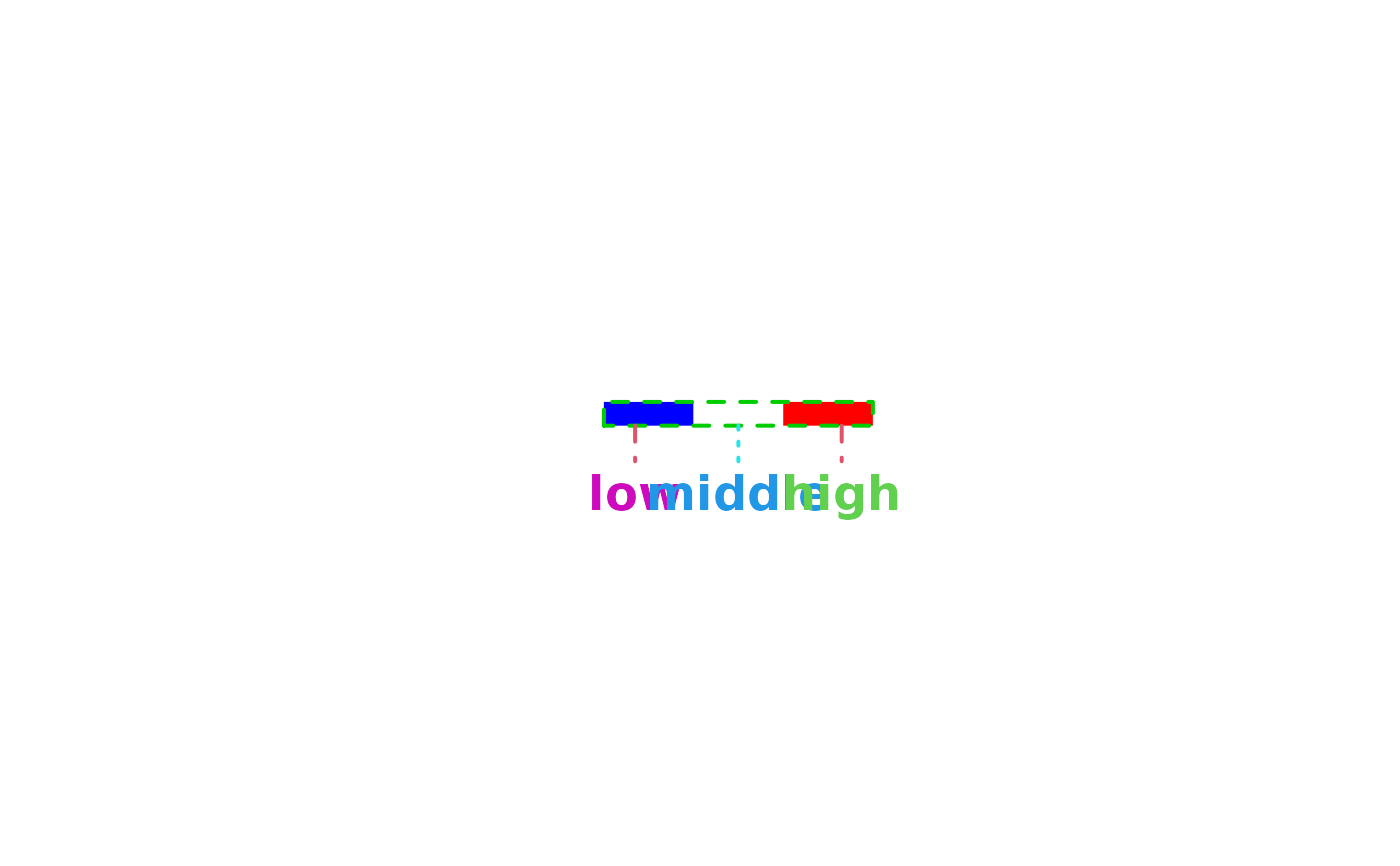colorlegend(color = c("red", "white", "red"), x = x, at = c(-1.5, 0, 1.5),
labels = c("low", "middle", "high"), length.ticks = 1.5,
lwd.border = 2, lwd.ticks = 2, cex.labels = 1.5, font = 2,
col.border = "green3", col.ticks = c(2, 5, 2),
col.labels = c(6, 4, 3), lty.border = 2, lty.ticks = c(2, 3, 2),
ncol = 3, breaks = c(-2, -1, 1, 2))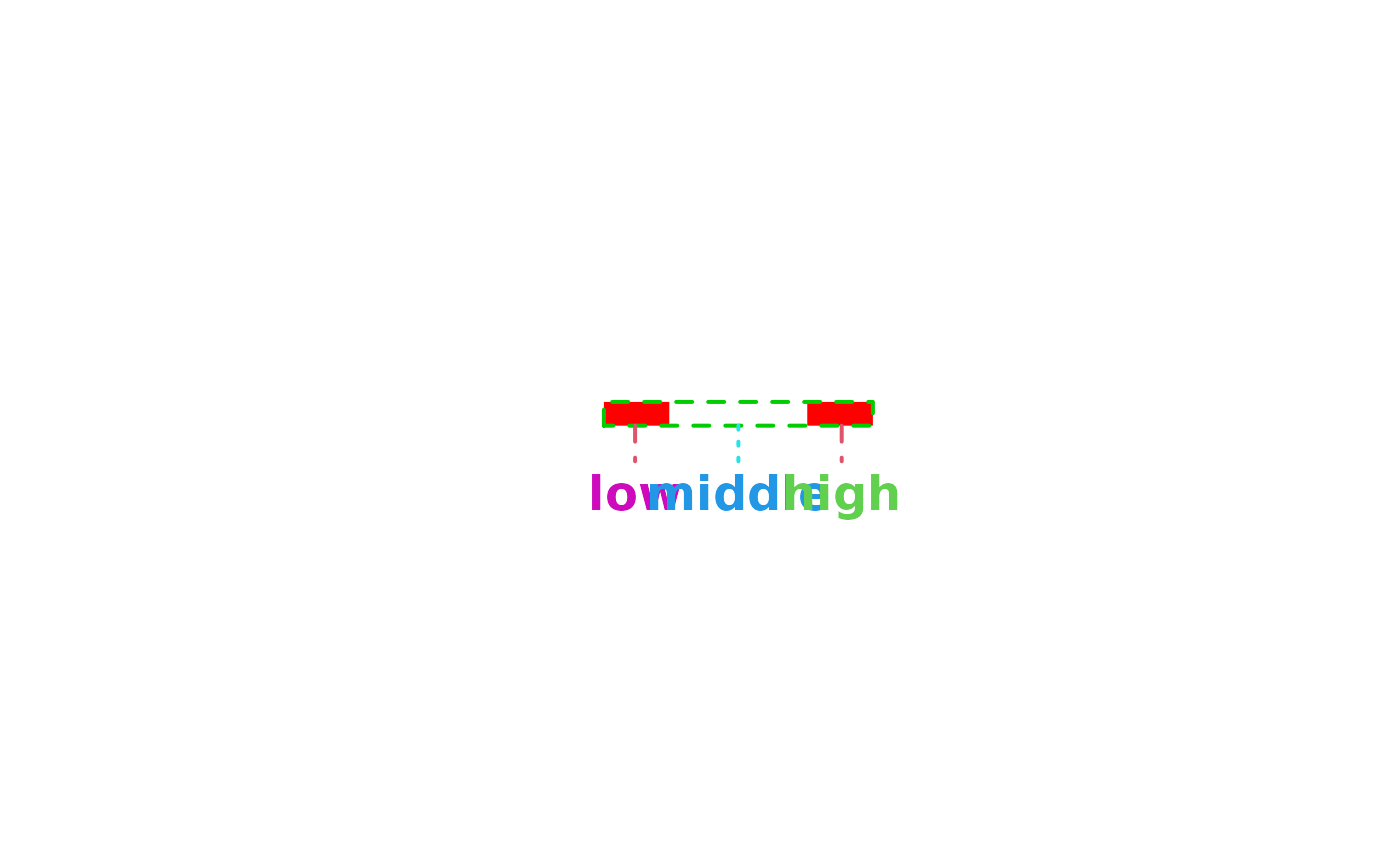colorlegend(color = diverge_hcl, x = x, range = c(-3, 3))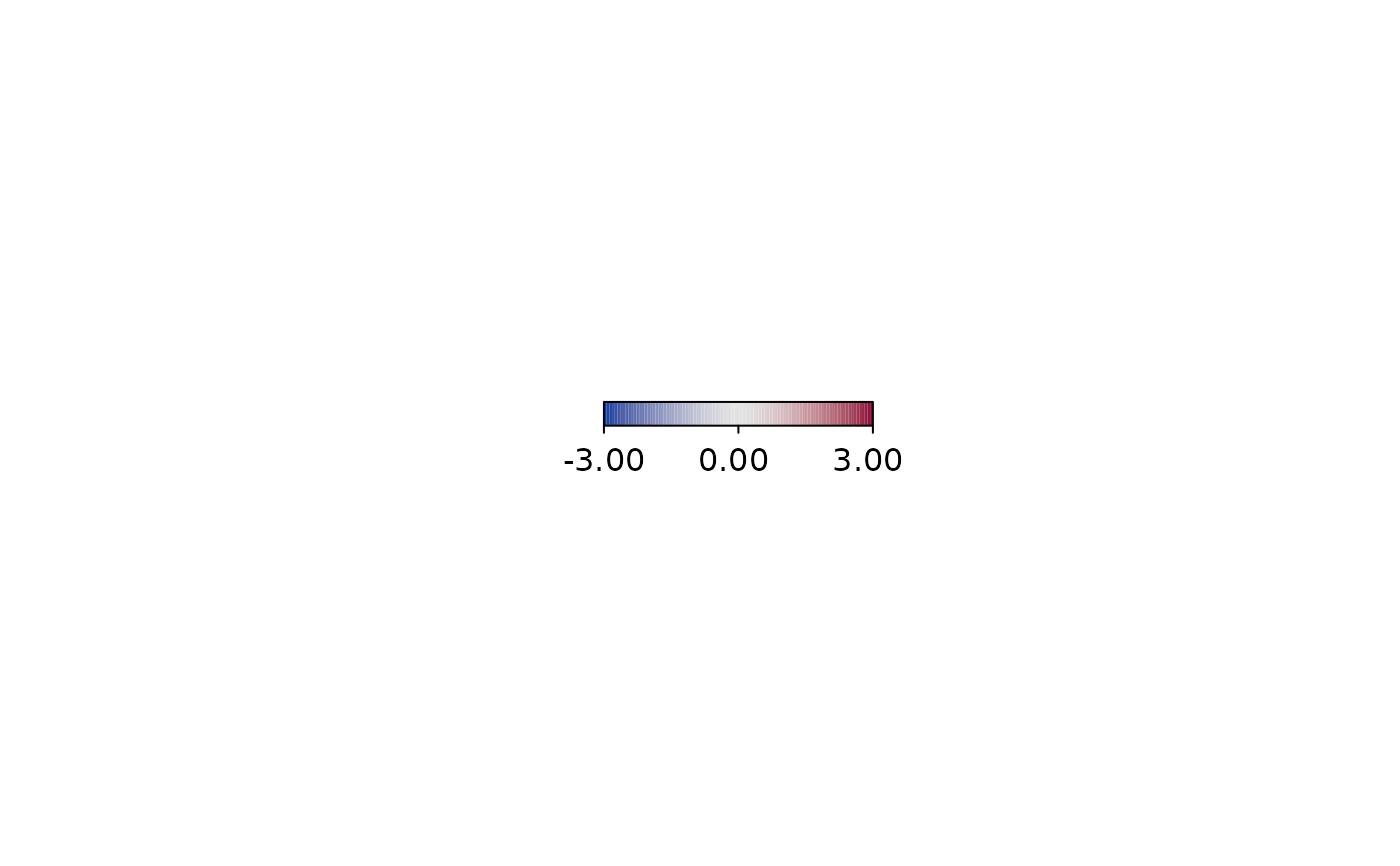colorlegend(color = diverge_hcl, x = x, range = c(-3, 3), lrange = c(-6, 6))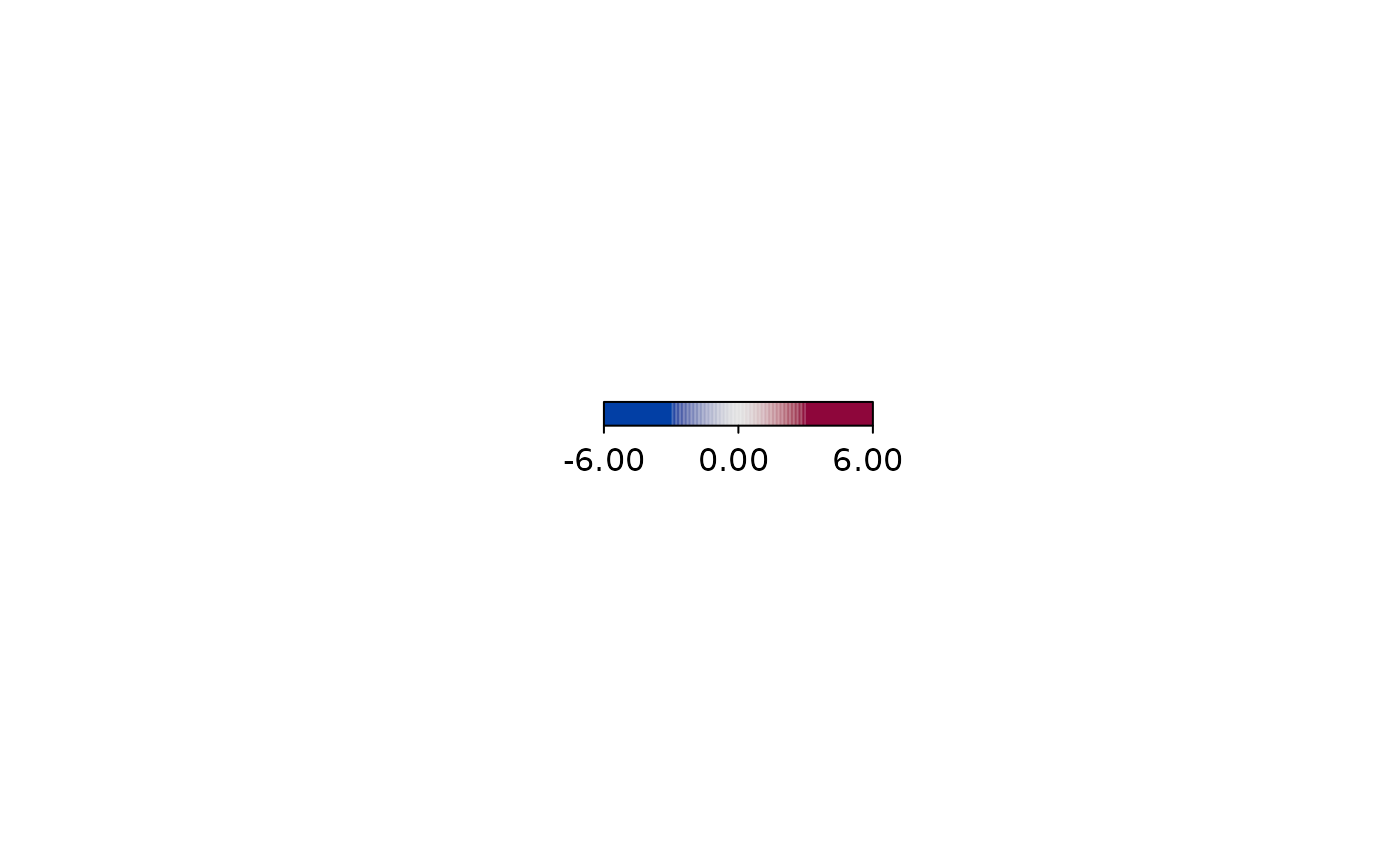## Combine plot with color legend.
n <- 100
x <- y <- seq(-3, 3, length.out = n)
z <- outer(sin(x), cos(x))
pal <- colorlegend(color = diverge_hcl, x = z, plot = FALSE)
par(mar = c(4.1, 4.1, 1.1, 1.1))
layout(matrix(c(1, 2), nrow = 1), widths = c(1, 0.3))
image(x = x, y = y, z = z, col = pal$colors, breaks = pal$breaks)
par(mar = c(4.1, 0.1, 1.1, 3.1))
colorlegend(color = diverge_hcl, x = z, plot = TRUE, full = TRUE,
side.legend = 2, side.ticks = 2)par(op)

## Another example with different plot.
n <- 50
x <- sin(seq(-3, 3, length.out = n))
pal <- colorlegend(color = diverge_hcl, x = x, plot = FALSE)
at = seq(min(x), max(x), length.out = 9))par(op)